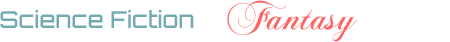## User post count & rep history

1

(click on this box to dismiss)Q&A for science fiction and fantasy enthusiasts

``````-- Return the number of post (Q/A separately) and the score of the post / 10
-- overtime

DECLARE @UserID Int = ##UserID?9449426##
DECLARE @RegisterDate Datetime
DECLARE @Now Datetime = GETDATE()

-- Create a table containing all the months since the user was registered to now
SELECT TOP 1 @RegisterDate = CreationDate
FROM Users
WHERE Id = @UserID

), 0) AS [ReportedMonth]
INTO    #Months
FROM    master.dbo.spt_values x
WHERE   x.type = 'P'
AND     x.number <= DATEDIFF(MONTH, @RegisterDate, @Now);

-- Get points from all user's posts
SELECT
Question = SUM(IIF(p.PostTypeId = 1,
CASE
WHEN v.VoteTypeId = 2 THEN 1
WHEN v.VoteTypeId = 3 THEN -0.2
ELSE 0
END,
0)),
CASE
WHEN v.VoteTypeId = 2 THEN 1
WHEN v.VoteTypeId = 3 THEN -0.2
ELSE 0
END,
0)),
Questions = 0,
DATEADD(m, DATEDIFF(m, 0, v.CreationDate), 0) [Month]
INTO #Score
FROM Posts p
JOIN Votes v on v.Postid = p.Id
WHERE v.VoteTypeId IN (2, 3)
AND p.PostTypeId IN (1, 2)
AND p.OwnerUserId = @UserID
GROUP BY DATEADD(m, DATEDIFF(m, 0, v.CreationDate), 0)

-- Get post count from all user's posts
SELECT
Question = 0,
Questions = SUM(IIF(p.PostTypeId = 1, 1, 0)),
Answers =   SUM(IIF(p.PostTypeId = 2, 1, 0)),
DATEADD(m, DATEDIFF(m, 0, p.CreationDate), 0) [Month]
INTO #PostCount
FROM Posts p
WHERE p.PostTypeId IN (1, 2)
AND p.OwnerUserId = @UserID
GROUP BY DATEADD(m, DATEDIFF(m, 0, p.CreationDate), 0)

SELECT *
INTO #Merged
FROM (
SELECT Question = 0, Answer = 0, Questions, Answers, [Month] FROM #PostCount
UNION ALL
SELECT Question, Answer, Questions = 0, Answers = 0, [Month] FROM #Score
) AS x

SELECT
ReportedMonth,
ISNULL(SUM(Questions) OVER (ORDER BY [ReportedMonth]), 0) [Num of Question],
ISNULL(SUM(Question)  OVER (ORDER BY [ReportedMonth]), 0) [Question Score],Hold tight while we fetch your results